# AN-1557: Implementing the AD5940 and AD8233 in a Full Bioelectric System

### Introduction

The AD5940 is a high precision, ultra low power, analog front end (AFE) system designed to excite and measure the current, voltage, or impedance response of a sensor.

The AD5940 features two high precision measurement loops. An ultra low power, sub 1 kHz loop and a high speed, sub 200 kHz loop allow the AD5940 to precisely measure the impedance of a sensor.

This application note details how to set up the AD5940 and the AD8233 in a full bioelectric system that can perform electrodermal activity (EDA), body impedance analysis (BIA), and electrocardiogram (ECG) measurements through the same set of electrodes.

The hardware used contains the AD5940 evaluation kit; which includes the EVAL-ADICUP3029 Arm® based Arduino form factor microcontroller; the EVAL-AD5940BIOZ evaluation board; and the AD5940 Z test board shown in Figure 1.

### Evaluation Kit

#### Evaluation Kit Contents

The AD5940 evaluation kit includes the following:

• ECG cable
• MicroUSB to USB cable

The EVAL-ADICUP3029 is the main motherboard for the evaluation kit. This board contains the on-board ADuCM3029 microcontroller. The ADuCM3029 is a leading edge Analog Devices, Inc., Arm Cortex M3 devices and is used to communicate to the AD5940 via the serial peripheral interface (SPI).

The EVAL-AD5940BIOZ board contains the AD5940 and the AD8233 chip used for ECG measurements. The EVAL-AD5940BIOZ is an Arduino Uno form factor board that plugs down onto the EVAL-ADICUP3029. This board contains the necessary discrete components required for ECG, EDA, and BIA measurements, including isolation capacitors and current limiting resistors. This board also features an interface to connect measurement cables and the AD5940 Z test board, which models skin and body impedance. For more information on the EVAL-AD5940BIOZ board, refer to the AD5940 User Guide wiki page.

The AD5940 Z test board contains a network of resistors, capacitors, and switches designed specifically for testing and verifying measurements. The AD5940 Z test board can model a range of body and skin impedances and plug into the EVAL-AD5940BIOZ board via the USB connector. For more information on the AD5940 Z test board, refer to the AD5940 User Guide wiki page.

ECG Cables

The ECG cables supplied with the evaluation kit are provided as a means of connecting the hardware to a human body simulator for ECG, BIA, and EDA measurements. Never connect the AD5940 evaluation board to the human body. Table 1 details the name and color mapping for the supplied cables.

 Electrode Name Color F+ Red S+ Green S− Blue F− Black

### Measurement System Overview

The AD5940 data acquisition loop consists of a low bandwidth loop, a high bandwidth loop, a high precision analog-to-digital converter (ADC), and a programmable switch matrix.

The low bandwidth loop consists of a low power, dual-output digital-to-analog converter (DAC) that generates the bias voltage for the low power transimpedance amplifier (TIA) (VZERO) and the bias voltage for the potentiostat amplifier (VBIAS).

The low bandwidth loop is used for low bandwidth signals where the frequency of the excitation signal is sub 200 Hz, such as EDA measurements.

The high bandwidth loop consists of a high speed DAC that is designed to generate a high frequency, ac excitation signal when making impedance measurements, and a high speed TIA designed to convert high bandwidth current signals up to 200 kHz into voltages measured by the ADC.

The high bandwidth loop is used for body impedance measurements where the excitation frequency is in the 50 kHz range.

The switch matrix is a series of programmable switches that allows the connection of external pins to the high speed DAC excitation amplifier and to the high speed TIA inverting input.

The switch matrix provides an interface to connect an external calibration resistor to the measurement system, as well as flexibility for connecting electrodes.

This application note describes how the AD5940 can be used in a software configurable bioelectric system with the AD8233. Through software, the system is capable of measuring BIA, EDA, or ECG on the same set of electrodes.

### 2-Wire Bioimpedance

#### Bioimpedance Measurement Theory

The AD5940 can take 2-wire bioimpedance measurements for general-purpose impedance measurements on the body or can measure internal body tissues.

2-wire bioimpedance is a voltammetry measurement. To measure an unknown impedance (ZUNKNOWN), an ac excitation the signal is applied across the unknown impedance. The voltage of the excitation signal is measured. Then, the current flow through the unknown impedance is measured. The current is converted to a voltage to be measured by the ADC via the TIA. A discrete Fourier transform (DFT) is performed on the ADC data for the current and voltage values. Using the real and imaginary parts, the magnitude is calculated to give the unknown impedance voltage (VZUNKNOWN) and unknown impedance current (IZUNKNOWN).

Use the following equation to calculate the unknown impedance magnitude of the sensor: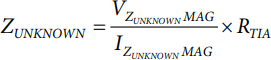where:
VZUNKNOWNMAG is the magnitude of the unknown impedance voltage.
IZUNKNOWNMAG is the magnitude of the unknown impedance current.
RTIA is the value of the high speed TIA gain resistor in Ω.

A bioimpedance measurement must conform to the IEC 60601 standard, which sets guidelines for the maximum allowable current that can enter the human body. The resistor limits (RLIMIT) the current. CISOx are the isolation capacitors that guarantee that no dc current enters the body. Guidelines for selecting the correct values can be found in the Calculations section.

#### Bioimpedance Solution Using the AD5940

This section describes the blocks of the AD5940 used to perform 2-wire bioimpedance measurements. As outlined in the Bioimpedance Measurement Theory section, a 2-wire bioimpedance measurement requires an ac voltage source, a voltage measurement channel, and a current measurement channel.

For 2-wire bioimpedance measurement, the impedance under test can be connected between the CE0 pin and SE0, DE0, or AINx. For the purposes of this application note, ZUNKNOWN is connected between CE0 and AIN1.

Excitation Signal

The AD5940 uses its waveform generator, high speed DAC, and excitation amplifier to generate high frequency excitation signals. The frequency is programmable from dc up to 200 kHz. The signal is applied to the sensor through the CE0 pin, as shown in Figure 3. The signal amplitude is programmable up to ±607 mV.

Measuring Voltage

The voltage of the excitation signal is measured as the voltage is applied to the sensor. The positive input to the ADC is the P node, which connects to CE0 via the switch matrix as shown in Figure 3. The negative input to the ADC is the N node, which connects to the negative input of the high speed TIA. Using the DFT hardware accelerator, a DFT is performed on the ADC data where the real and imaginary parts are calculated and stored in the data first-in, first-out (FIFO).

Measuring ZUNKNOWN Current

To measure the ZUNKNOWN current, the same excitation signal is applied to the sensor. The high speed TIA converts the current to a voltage to be read by the ADC via the gain resistor, RTIA. Similarly, a DFT is performed on the ADC results, and the real and imaginary parts are stored in the data FIFO to be read by the host microcontroller.

Calculating ZUKNOWN

The AD5940 uses the sequencer to store commands. A host microcontroller (in this case, the EVAL-ADICUP3029) writes the required commands to the sequencer. These commands set up the DAC, ADC, and TIA for measurements. The sequencer executes the commands automatically, independent of the microcontroller. The voltage measurement is performed first. An interrupt is generated when the data FIFO is full. The microcontroller then reads the FIFO and stores the real and imaginary DFT results. The ADC mux is then configured to connect the high speed TIA output to the ADC to measure current. The sequence is rerun and the AD5940 generates an interrupt when the data FIFO is full, which alerts the host controller to read the data.

Use the following equations to determine ZUNKNOWN: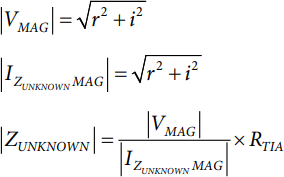where:
VMAG is the magnitude of the voltage across the sensor.
r and i are the real and imaginary components from the current and voltage DFT measurements.

#### Calculations

To optimize the AD5940 for 2-wire bioimpedance measurements, calculate the RLIMIT value, add isolation capacitors (RTIA), and calculate the precision resistor (RCAL) value.

Calculate RLIMIT

To conform to IEC 60601 standards, limit the amount of ac current entering the human body. The maximum allowable ac current at is 500 µA at 50 kHz and is 600 µA at 60 kHz. When calculating the RLIMIT resistor value, the maximum output voltage from the AD5940 is 1.2 V p-p (0.4243 V rms). Set the maximum allowable ac current to 80% of maximum, or 400 µA rms. The following equation is the result of these values:As such, a 1 kΩ RLIMIT is selected and is connected to the CE0 pin on the AD5940. This calculation ignores CISOx because of its small size.

Isolation Capacitors

To conform to IEC 60601 standards, a 10 µA maximum dc current is allowed to enter the human body. In this application, the dc current is guaranteed to be zero due to the addition of isolation capacitors. A value of 0.47 µF is selected for the isolation capacitors because 0.47 µF is a sufficiently large capacitance that is also available in small packages suitable for wearable electronics. CISO1, the isolation capacitor on CE0, is modified to 15 nF so the capacitor is suitable for EDA and BIA measurements. See the EDA section for details on calculation.

Calculating RTIA

To calculate RTIA, the gain resistor for the high speed TIA, first calculate the minimum impedance and maximum current of the high speed TIA. In the following equation, RACCESS1MIN and RACCESS2MIN represent resistances in leads connecting the sensor and are assumed 0 Ω. Solving the equation gives a ZMIN = 1.2 kΩ.where:
ZMIN is the minimum impedance.
ZUNKNOWNMIN is the minimum unknown impedance. Assumed to be 100 Ω in this equation.
XCISO1MIN and XCISO2MIN are 67.73 Ω at 50 kHz.

The maximum output voltage from the high speed DAC is 600 mV peak. Calculate the maximum current (IMAX) into the TIA as follows:where ZMIN is the minimum impedance.

The peak voltage allowed at the TIA output is 900 mV peak when the ADC programmable gain amplifier (PGA) gain is 1 or 1.5. As such,There is no 1.8 kΩ RTIA option on the AD5940. Therefore, the 1 kΩ option was selected.

Selecting RCAL

RCAL is a precision resistor used in conjunction with the high speed DAC and excitation amplifier to generate accurate currents. RCAL calibrates the high speed TIA gain resistor. Optimally, select an RCAL value close to that of RTIA. In this case, RCAL must be 1 kΩ. To guarantee accuracy, the resistor must have a 0.1% tolerance.

Calculating ZUNKNOWN

The AD5940 uses the sequencer to store commands. A host microcontroller writes the required commands into command memory, and the AD5940 executes the commands automatically. The first measurement taken is the voltage measurement. It is only necessary to measure the voltage once because the result is stored. The sequencer then runs the measurement sequence which applies the excitation voltage and measures the response current performing a DFT on the data. The real and imaginary parts are stored in the FIFO. The sequencer can be configured to run periodically using the sleep and wakeup timer.

The host microcontroller reads the data FIFO to get the real and imaginary results for the current measurement. Using these values, the following equations determine the voltage measurement magnitude and voltage measurement phase:To calculate the impedance, use Ohm’s law by dividing the voltage magnitude by the current magnitude. Convert the current measurement value into a voltage using RTIA. This gain must be taken into account. Therefore, the equation to determine the unknown impedance is as follows:#### Taking 2-Wire Bioimpedance Measurements

Hardware Setup

The EVAL-ADICUP3029, EVAL-AD5940BIOZ, and the AD5940 Z test board are required to perform 2-wire bioimpedance measurements. Stack the boards as shown in Figure 1.

On the AD5940 Z test board, there are five switch banks, S1 to S5. Bank S2 and Bank S4 simulate contact and lead impedances. S1 simulates bioimpedance. Note that S3 and S5 are not applicable to 2-wire bioimpedance measurements. To test measurement accuracy,set all switches on S2 and S4 to the on position, which sets the contact and lead impedance to zero. Toggle Switch 7 on Bank S1 to set the unknown impedance value to 2 kΩ, as shown in Figure 4.

Firmware Setup

The AD5940 software development kit has a dedicated 2-wire bioimpedance measurement example. To run the BIA measurements on the evaluation hardware, the user can use the Analog Devices SensorPal graphical user interface (GUI) tool (see the AD5940) or the IAR Embedded Workbench firmware example.

For quick prototyping, use the SensorPal tool. SensorPal provides a number of configurable parameters to define the measurement, as well as a quick graphing mechanism.

Alternatively, navigate to the Examples folder in the software development kit and select AD5940_BIOZ-2WIRE > ADICUP3029 > ADICUP3029.eww. Open the ADICUP3029.eww file to open the project workspace in IAR Embedded Workbench.

To run the project, take the following steps:

1. Compile and build the project.
2. Start the debugger to begin code execution.
3. Open a terminal program such as RealTerm and configure the baud rate to 230400.
4. Select the communications port (COM) to which the EVAL-ADICUP3029 is connected.

The measurement results are streamed over a universal asynchronous receiver transmitter (UART) and can be saved to a file for analysis.

To modify the default application parameters, use the AD5940BIOZStructInit(void) function in the AD5940Main.c file. The AppBIOZCfg_Type data structure contains the configurable parameters for the application. For more details on the firmware, refer to the AD5940_Library_and_examples.chm file located in the doc folder in the software development kit.

Measurement Results

The measurement results displayed on the terminal are shown in Figure 6. The magnitude is approximately 4162 Ω because the 2-wire bioimpedance measurement measures the impedance under test, the contact impedance, the current limiting resistors, and the isolation capacitors.

For Figure 7,where:
RzMag is the magnitude, or R1||R2 + C1.
RLIMIT1 is a current limiting resistor to comply with IEC 60601.
XCISO1 is the reactance of CISO1.
REC1 is the contact impedance.
R1 is used to model bioimpedance.
R2 is used to model bioimpedance.
REC2 is the contact impedance.
XCISO2 is the reactance of CISO2.
RLIMIT2 is a current limiting resistor to comply with IEC 60601.

The Impedance Profiling_2wireIEC.xls workbook provides equations for calculating expected results, taking into account the limiting resistors, isolation capacitors, and contact impedances.

On the EVAL-AD5940BIOZ evaluation board, RLIMIT1 and RLIMIT2 are both 1 kΩ, CISO1 is 15 nF, and CISO2 is 470 nF. Assume that REC1 is 0. By entering these values into the workbook (as in Figure 8), the theoretical values match closely with the measured values. The small error is the result of the tolerances of the components.

### ECG

#### ECG Measurement Theory

An ECG measures how the electrical activity of the heart changes over time as action potentials propagate throughout the heart during each cardiac cycle. An ECG does not directly measure the cellular depolarization and repolarization within the heart but rather the relative, cumulative magnitude of populations of cells eliciting changes in their membrane potentials at a given point in time. An ECG shows electrical differences across the heart when depolarization and repolarization of these atrial and ventricular cells occur.

Typically, the ECG is performed by placing two electrodes directly on the skin and reading the potential difference between the electrodes. This method is possible because these signals are transmitted throughout the body. The detected waveform features depend not only on the amount of cardiac tissue involved but also on the orientation of the electrodes with respect to the dipole in the heart. The ECG waveform looks slightly different when measured from different electrode positions, and typically, an ECG is obtained using a number of different electrode locations (such as limb leads or precordial) or configurations (such as unipolar, bipolar, and modified bipolar).

For the purposes of an ECG, consider the human body as a large volume conductor. The human body is filled with tissues surrounded by a conductive ionic fluid. The heart is suspended inside of that conductive medium. During the cardiac cycle, the heart contracts in response to action potentials moving along the chambers of the heart.Figure 9. Typical ECG Waveform Compared to the Timing of Atrioventricular and Semilunar Valve Activity, Segments of the Cardiac Cycle During Which the Ventricles Are in Systole or Diastole.

This section describes how to use the AD5940 and the AD8233 to take ECG measurements. Connect the four measurement electrodes to the switch matrix of the AD5940. Figure 10 highlights the signal chain for ECG measurements. Connect Electrode E4 to the right leg drive (RLD) input of the AD8233 directly. Join E1 and E2 by closing internal switches on the AD5940 switch matrix and connecting to the AD8233 IN+ input via AFE2 by closing Switch P5 and Switch P6. Connect E3 to the AD8233 via AIN0 and AFE3 by closing Internal Switch N7 and Internal Switch N1. Connect the output of the AD8233 to Auxiliary Input AIN6 on the AD5940.

The AD5940 uses the sequencer to store measurement commands. A host microcontroller (in this case, the ADuCM3029) writes the required commands to the command memory. The sequencer runs the commands independent of the microcontroller. The initialization sequence is run first to configure the voltage references, the switch matrix, the ADC input source, and the ADC filters. The measurement sequence runs periodically with a user defined sample frequency to sample ECG data from the AD8233 on the AIN6 pin.

#### Taking ECG Measurements

Hardware Setup

The EVAL-AD5940BIOZ board included in the evaluation kit is ready to use (no hardware modifications are required). There are a number of surface-mount resistors and capacitors connected to AD8233 pins that set the system bandwidth. On the EVAL-AD5940BIOZ board, the components were selected for monitor bandwidth (0.34 Hz to 38 Hz) with a system gain of 296. To calculate the appropriate passive values for different cutoff frequencies, download the AD8232_Filter_Design_Tool.zip file found on the AD8232 product page.

This sport bandwidth (7 Hz to 21 Hz) is intended for nonclinical applications such as a healthcare watch where ECG peaks are of interest. For applications where other aspects of the ECG waveform (such as the P wave, Q wave, S wave, or T wave) are of interest, the monitor bandwidth is required.

The AD5940 evaluation kit is equipped with custom ECG cables. These cables can connect to an ECG simulator for testing the hardware. Connect the RLD electrode to F−. Connect the right hand (RH) to F+ and S+. Connect the left hand (LH) to S−, as shown in Figure 11.

Never connect the EVAL-AD5940BIOZ evaluation board to the human body.

Firmware Setup

The AD5940 software development kit has a dedicated ECG measurement example. To run the ECG measurements on the evaluation hardware, the user can use the Analog Devices SensorPal GUI tool or the IAR Embedded Workbench firmware example.

For quick prototyping, use the SensorPal tool. SensorPal provides a number of configurable parameters to define the measurement, as well as a quick graphing mechanism.

Alternatively, navigate to the Examples folder in the software development kit and select AD5940_ECG > ADICUP3029 > ADICUP3029.eww. Open the ADICUP3029.eww file to open the project workspace in IAR Embedded Workbench.

To compile and run the project, perform the following steps:

1. Compile and build the project (refer to the AD5940 User Guide wiki page).
2. Start the debugger to begin code execution.
3. Open a terminal program such as RealTerm and configure the baud rate to 230400.
4. Select the COM port to which the EVAL-ADICUP3029 will be connected.

The measurement results are then streamed over a UART and can be saved to a file for analysis and to create graphs.

To modify the default application parameters, use the AD5940ECGStructInit(void) function. The AppECGCfg_Type data structure contains the configurable parameters for the application. For more details on the firmware, refer to the AD5940_Library_and_examples.chm file located in the doc folder in the software development kit.

### 4-Wire BIA

#### 4-Wire BIA Measurement Theory

The 4-wire BIA approach uses a high precision, ac voltage source to excite a sensor with a known ac voltage (VAC). Simultaneously, a common-mode voltage is applied across the sensor. To calculate the impedance, measure the current (I) that flows from the unknown impedance and the voltage across the unknown impedance.

Calculate the impedance by using the following equation:In real-world applications, medical devices must conform to the IEC 60601 standard. This standard limits the amount of dc and ac voltage that can be applied to the human body. In Figure 14, there are discrete isolation capacitors (CISO1, CISO2, CISO3, and CISO4) that ensure no dc voltage occurs across the body. RLIMIT limits the current provided to the sensor to conform to the IEC 60601 standard. RACCESSx represents the resistances of the electrodes connecting to the unknown impedance.

#### 4-Wire BIA Solution Using the AD5940

As outlined in the 4-Wire BIA Measurement Theory section, a 4-wire bioimpedance solution requires a precision ac voltage source, a high precision current meter, and a precision differential voltage meter.

The Precision AC Voltage Source section, the High Precision Current Meter section, and the Precision Differential Voltage Meter section describe how these components are implemented on the AD5940.

Precision AC Voltage Source

The AD5940 uses a high speed DAC and waveform generator to generate the precision ac voltage. An internal differential sense configuration guarantees the accuracy of the voltage source by connecting CE0 and AIN1 back into the excitation buffer. Switch D5 in the programmable switch matrix connects the output of the excitation loop to the CE0 pin connected to the sensor. A sine wave generator generates a sine wave and outputs through the 12-bit, high speed DAC and excitation amplifier.

High Precision Current Meter

The AD5940 uses a high speed, high precision TIA for converting current from the sensor into a voltage measured by the ADC (see Figure 15). The TIA channel measures the response current and is precisely biased by the internal 1.11 V source. Tie the T channel and the N channel together using the switch matrix for accurate sense capability on the current measured. Figure 15 shows the connection between the sensor, the high speed TIA, and ADC of the AD5940. In Figure 15, HSTIA_P is the mux signal from the high speed TIA into the ADC mux. The ADC converts the current measurement with an 800 kSPS speed. A DFT is performed on the data. The DFT is implemented on the AD5940. The number of DFT points is configurable up to 16,384. The AD5940 calculates the real and imaginary parts, and the host microcontroller calculates the unknown impedance of the sensor.

Precision Differential Voltage Meter

The AD5940 uses a low power DAC and a low power TIA to set the common-mode voltage between AIN2 and AIN3/BUF_VREF1V8 through the AIN4/LPF0 pin. Connect the VBIAS output of the low power DAC to the low power TIA positive input. The low power DAC is configured to output a precise 1.1 V. Connect the internal RFILTER resistor at the low power TIA output. Connect the common-mode voltage (VCM) to AIN4/LPF0, which has a capacitor to GND that creates a low-pass filter.

#### Calculating Discrete Components for BIA

There are a number of discrete components needed in the system to guarantee safety and accuracy. This section describes the calculations used to select suitable isolation capacitors, gain resistors for the high speed TIA, and a current-limit resistor.

Current Limit Resistor

To conform to IEC 60601 standards, limit the amount of ac current entering the human body. The maximum allowable ac current at is 500 µA at 50 kHz and is 600 µA at 60 kHz. When calculating the RLIMIT resistor value, the maximum output voltage from the AD5940 is 1.2 V p-p (0.4243 V rms). Set the maximum allowable ac current to 80% of maximum, or 400 µA rms. The following equation is the result of these values:As such, a 1 kΩ RLIMIT is selected and is connected to the CE0 pin on the AD5940. This calculation ignores CISOx because of its small size.

Isolation Capacitors

To conform to IEC 60601 standards, a 10 µA maximum dc current is allowed to enter the human body. In this application, the dc current is guaranteed to be zero due to the addition of isolation capacitors. A value of 0.47 µF is selected for the isolation capacitors because 0.47 µF is a sufficiently large capacitance that is also available in small packages suitable for wearable electronics. CISO1, the isolation capacitor on CE0, is modified to 15 nF so the capacitor is suitable for EDA and BIA measurements. See the EDA section for details on calculation.

High Speed TIA Gain Resistor

To calculate RTIA, the gain resistor for the high speed TIA, first calculate the minimum impedance and maximum current of the high speed TIA. In the following equation, RACCESS1MIN and RACCESS2MIN represent resistances in leads connecting the sensor and are assumed 0 Ω. Solving the equation gives a ZMIN = 1.2 kΩ.where:
ZUNKNOWNMIN is assumed to be 200 Ω in this equation.
XCISO1MIN and XCISO2MIN are 67.73 Ω at 50 kHz.

The maximum voltage is 600 mV peak. The maximum current into TIA = 600 mV and into ZMIN = 500 μA. The peak voltage at output of TIA = 900 mV peak, which is within the ADC range. As such, RTIA = 900 mV peak (500 μA peak) = 1.8 kΩ. There is no 1.8 kΩ. RTIA option on the AD5940. Therefore, the 1 kΩ option was selected.

Calculating ZUNKNOWN

The AD5940 uses the sequencer to store commands. A host microcontroller writes the required commands into command memory, and the AD5940 executes the commands automatically. The sequencer runs the commands and fills the data FIFO with the DFT real and imaginary results for both the voltage and current measurements (four data points in total).

The host microcontroller reads the data FIFO and uses the real and imaginary DFT results to calculate the unknown impedance. Calculate the impedance of the sensor by using the following equations: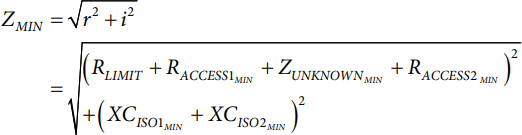To calculate the impedance, use Ohm’s law by dividing the voltage magnitude by the current magnitude. Convert the current measurement value into a voltage using RTIA. This gain must be taken into account. Therefore, the equation to determine the unknown impedance is as follows:#### Taking BIA Measurements

Hardware Setup

The EVAL-ADICUP3029, EVAL-AD5940BIOZ, and the AD5940 Z test board are required to perform BIA measurements. Stack the boards as shown in Figure 1.

The jumpers on the EVAL-AD5940BIOZ board do not have any impact on BIA measurements and can remain in the default position.

On the AD5940 Z test board, there are five switch banks S1 to S5. S2, S3, S4, and S5 are used to simulate contact and lead impedances. S1 is used to simulate body impedance. To test measurement accuracy, set all switches on S2 to S4 to the on position, which sets the contact and lead impedance to zero. Open Switch 9 on Bank S1 to set the unknown impedance value to 2 kΩ, as shown in Figure 16.

Firmware Setup

The AD5940 software development kit has a dedicated BIA measurement example. To run the BIA measurements on the evaluation hardware, the user can use the Analog Devices SensorPal GUI tool or the IAR Embedded Workbench firmware example.

For quick prototyping, use the SensorPal tool. SensorPal provides a number of configurable parameters to define the measurement, as well as a quick graphing mechanism.

Alternatively, navigate to the Examples folder in the software development kit and select AD5940_BIA > ADICUP3029 > ADICUP3029.eww. Open the ADICUP3029.eww file to open the project workspace in IAR Embedded Workbench.

To run the project, perform the following steps:

1. Compile and build the project.
2. Start the debugger to begin code execution.
3. Open a terminal program such as RealTerm and configure the baud rate to 230400.
4. Select the COM port to which the EVAL-ADICUP3029 will be connected.

The measurement results are streamed over a UART and can be saved to a file for analysis.

To modify the default application parameters, use the AD5940BIAStructInit(void) function. The AppBIACfg_Type data structure contains the configurable parameters for the application. For more details on the firmware, refer to the AD5940_Library_and_examples.chm file located in the doc folder in the software development kit.

### EDA

#### EDA Measurement Theory

EDA is a voltammetry measurement. To measure an unknown impedance, an ac excitation signal is applied across the unknown impedance. The voltage across the unknown impedance is measured. Then, the current flow through the unknown impedance is measured. The current is converted to a voltage to be measured by the ADC via the TIA. A DFT is performed on the ADC data for the current and voltage values. Using the real and imaginary parts, the magnitude is calculated to give VZUNKNOWN and IZUNKNOWN.

Use the following equation to calculate the unknown impedance magnitude of the sensor: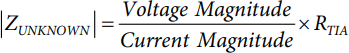#### EDA Solution Using the AD5940

This section describes the blocks of the AD5940 used to perform EDA impedance measurements. As outlined in the EDA Measurement Theory section, an EDA measurement requires an excitation voltage, a measurement of VZUNKNOWN, and a measurement of the ZUNKNOWN current.

Excitation Signal

The AD5940 uses its waveform generator and low power DAC to generate a low frequency sine wave (≈ 100 Hz). The sine wave is applied to the sensor via the potentiostat amplifier connected to the CE0 pin (as shown in Figure 21).

Measuring Voltage

Connect the unknown impedance between the CE0 pin and the SE0 pin. Connect SE0 to the inverting input of the low power TIA. Connect a high precision reference to the TIA to maintain a common mode. To measure the voltage across ZUNKNOWN, measure the voltage on CE0 and select the voltage on the CE0 pin (VCE0) as the input to the ADC. The measurement sequence then initiates, and a DFT is performed on the measured data. Real and imaginary parts are stored in the data FIFO and are read by the host controller.

Measuring ZUNKNOWN Current

To measure the ZUNKNOWN current, use the same setup used to measure voltage. However, in this case, the current is measured through the TIA. Select the low-pass filter output (LPTIA_LPF0) as the input to the ADC. Rerun the measurement sequence, and a DFT is then performed on the ADC data. The real and imaginary parts are stored in the data FIFO and are read by the host controller.

Calculating ZUKNOWN

The AD5940 uses the sequencer to store commands. A host microcontroller (in this case, the EVAL-ADICUP3029) writes the required commands to the sequencer. These commands set up the DAC, ADC, and TIA for measurements. The sequencer executes the commands automatically, independent of the microcontroller. The voltage measurement is performed first. An interrupt is generated when the data FIFO is full. The microcontroller then reads the FIFO and stores the real and imaginary DFT results. The ADC mux is then configured to connect the low power TIA, low-pass filter output to the ADC to measure current. The sequence is rerun, and the AD5940 generates an interrupt when the data FIFO is full, which alerts the host controller to read the data.

Use the following equations to determine ZUNKNOWN:where r and i are the real and imaginary components from the current and voltage DFT measurements.

#### Calculating Discrete Components for EDA

There are a number of discrete components needed in the system to guarantee safety and accuracy. This section describes the calculations used to select a suitable current-limit resistor and isolation capacitors.

Calculating Limit Resistor

An 1 kΩ RLIMIT is used for BIA measurement. Because both measure-ments share the same electrode, RLIMIT for EDA is also 1 kΩ.

Isolation Capacitors

CISO2, the capacitor on the return path for EDA, is 0.47 µA to match the BIA measurement. Select a suitable CISO1 value to ensure that the ac current in the human body does not exceed 10 µA in the worst case.

See Figure 20 for worst case conditions, where resistor body = 0 Ω and capacitor body is a short circuit. Under these conditions, the largest current flows in the circuit. Use the following equations to calculate a suitable isolation for the EDA application.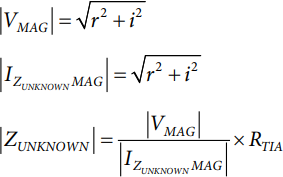where:
IACRMSLIMIT is the maximum allowed ac current in the human body.
IEXCRMS = 1.1 VPEAK/√2, where 1.1 V is the maximum range of the low power DAC.
fEXC is 100 Hz.

To find the maximum value for CISO1, redistribute the previous two equations, as follows: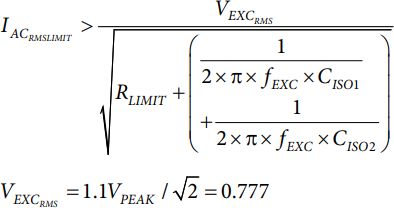Consider the worst case tolerances for the resistors and capacitors, as follows:

• 1% for RLIMIT
• 5% for CISO2
• 20% for CISO1
• 10% for fEXC

With these values, the equation to calculate CISO1 is as follows:Where CISO1 < 14 nF.

#### Minimizing Power Consumption for Always on Application

The EDA use case takes advantages of a key power saving feature of the AD5940. The AD5940 has a feature where the low power loop can run off the 32 kHz clock. After waking up from hibernate, the AD5940 system clock is switched to the 32 kHz oscillator (LPMODECLKSEL Bit 0 = 0x1). The waveform generator, low power DAC, ADC, and DFT engine run on the 32 kHz oscillator. All data and DFT results are captured in this mode, minimizing the current consumption of the AD5940. At the end of the measurement sequence, the AD5940 switches back to the 16 MHz clock, disables all blocks, and returns to hibernate. With a sample rate of 4 Hz and a DFT number of 16, the total average current consumption of the AD5940 is 66 µA.

When the AD5940 is running on the 32 kHz oscillator, it is not possible to communicate with the device through the SPI interface. Ensure that the SPI transaction occurs at the correct time. Figure 22 shows the measurement timeline for an EDA measurement with a sample rate of 4 Hz. When the measurement is completed, the host microcontroller can read back the data in a 200 ms window. Ensure that thislimit is not violated.

#### Taking EDA Measurements

Hardware Setup

The default jumper settings on the EVAL-AD5940BIOZ have no bearing on EDA measurement and can be left as is.

On the AD5940 Z test board, Bank S2 and Bank S3 are used for EDA measurements. Remove the P1 jumper, and close S9.

Firmware Setup

The AD5940 software development kit has a dedicated EDA measurement example. To run EDA measurements on the evaluation hardware, the user can use the SensorPal GUI tool or the IAR Embedded Workbench firmware example.

For quick prototyping, use the SensorPal tool. SensorPal provides a number of configurable parameters to define the measurement, as well as a quick graphing mechanism.

Alternatively, navigate to the Examples folder in the software development kit and select AD5940_EDA > ADICUP3029 > ADICUP3029.eww. Open the ADICUP3029.eww file to open the project workspace in IAR Embedded Workbench.

To run the project, perform the following steps:

1. Compile and build the project.
2. Start the debugger to begin code execution.
3. Open a terminal program such as RealTerm and configure the baud rate to 230400.
4. Select the COM port to which the EVAL-ADICUP3029 is connected.

The measurement results are streamed over a UART and can be saved to a file for analysis.

To modify the default application parameters, use the AD5940EDAStructInit(void) function. The AppEDACfg_Type data structure contains the configurable parameters for the application. For more details on the firmware, refer to the AD5940_Library_and_examples.chm file located in the doc folder in the software development kit.